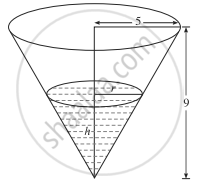Advertisement Remove all ads

# A right circular cone has height 9 cm and radius of the base 5 cm. It is inverted and water is poured into it. If at any instant the water level rises at the rate of - Mathematics and Statistics

Sum

A right circular cone has height 9 cm and radius of the base 5 cm. It is inverted and water is poured into it. If at any instant the water level rises at the rate of (pi/"A")cm/sec, where A is the area of the water surface A at that instant, show that the vessel will be full in 75 seconds.

Advertisement Remove all ads

#### SolutionLet r be the radius of the water surface and h be the height of the water at time t.

∴ area of the water surface A = πr2 sq cm.

Since the height of the right circular cone is 9 cm and radius of the base is 5 cm.

"r"/"h" = 5/9    ∴ r = 5/9"h"

∴ area of water surface, i.e. A = pi(5/9 "h")^2

∴ A = (25 pi"h"^2)/81      .....(1)

The water level, i.e. the rate of change of h is "dh"/"dt" rises at the rate of (pi/"A") cm/sec.

∴ "dh"/"dt" = pi/"A" = (pi xx 81)/(25 pi "h"^2)    ....[By (1)]

∴ "dh"/"dt" = 81/(25 "h"^2)

∴ "h"^2 "dh" = 81/25 "dt"

On integrating, we get

int "h"^2 "dh" = 81/25 int "dt" + "c"

∴ "h"^3/3 = 81/25 * "t + c"

Initially, i.e. when t = 0, h = 0

∴ 0 = 0 + c           ∴ c = 0

∴ "h"^3/3 = 81/25 "t"

When the vessel will be full, h = 9

∴ (9)^3/3 = 81/25 xx "t"

∴ t = (81 xx 9 xx 25)/(3 xx 81) = 75

Hence, the vessel will be full in 75 seconds.

Is there an error in this question or solution?
Advertisement Remove all ads

#### APPEARS IN

Advertisement Remove all ads
Advertisement Remove all ads
Share
Notifications

View all notifications

Forgot password?